## 用R语言解读凯利公式

• 张丹(Conan), 程序员R,Nodejs,Java
• weibo：@Conan_Z
• blog: http://blog.fens.me
• email: bsspirit@gmail.com1. 开始赌局
2. 凯利公式
3. 赌局的最优解
4. 让时间帮我们赚钱

## 1. 开始赌局

``````
# 设置胜负，1胜，0负
> win<-c(1,1,1,0,1,1,0,1,1,1)

# 分别按投注计算每回合的剩余资金
> a1<-(1+0.9)*100
> a2<-a1*(1+0.9)
> a3<-a2*(1+0.9)
> a4<-a3*0.1
> a5<-a4*(1+0.9)
> a6<-a5*(1+0.9)
> a7<-a6*0.1
> a8<-a7*(1+0.9)
> a9<-a8*(1+0.9)
> a10<-a9*(1+0.9)

> dat<-c(a1,a2,a3,a4,a5,a6,a7,a8,a9,a10)
> df<-data.frame(win,dat)

# 打印剩余资金列表
> df
win      dat
1    1 190.0000
2    1 361.0000
3    1 685.9000
4    0  68.5900
5    1 130.3210
6    1 247.6099
7    0  24.7610
8    1  47.0459
9    1  89.3872
10   1 169.8356
``````

10次交易后，赢了8次，只输了2次，我们从100元本金，上升到了169元，收益率为69%，还是不错的。最高的时候，资金为685元，收益率为685%，赚了6倍多。最低则是只剩下24元，真是赔的好惨啊！

``````
# 画出资金曲线
> plot(df\$dat,type='l')
``````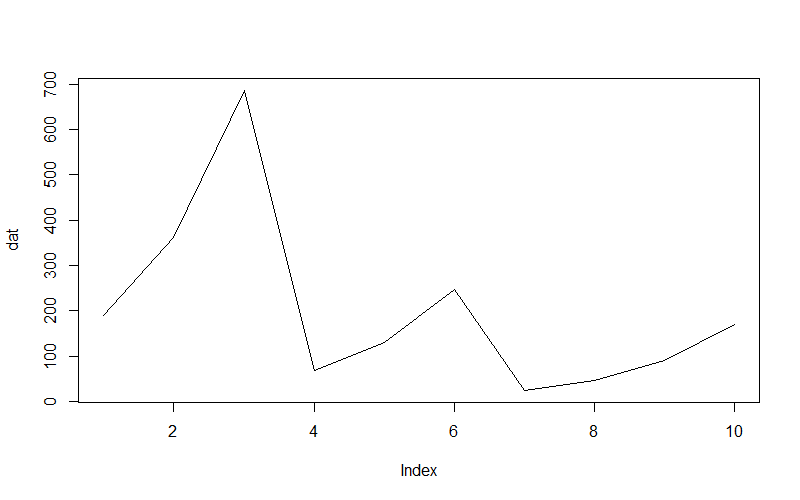``````
> library(magrittr)

# 定义现金流量函数：win=胜负结果,b=赔率,pos=仓位
> postion<-function(win,b=1,pos=0.6){            # 省略代码
+ }

# 设置胜负，1胜，0负
> win<-c(1,1,1,0,1,1,0,1,1,1)
> prob<-0.8                      # 胜率
> n<-10                          # 赌局数
> b<-1                           # 赔率
> caption<-100                   # 金额

# 分别计算不同仓位的剩余现金
> pos90<-postion(win,b,0.9)*caption
> pos60<-postion(win,b,0.6)*caption
> pos40<-postion(win,b,0.4)*caption
> pos20<-postion(win,b,0.2)*caption
> pos10<-postion(win,b,0.1)*caption

# 合并到数据框
> df1<-data.frame(win,pos90,pos60,pos40,pos20,pos10)

# 打印计算结果
> df1
win    pos90   pos60   pos40   pos20   pos10
1    1 190.0000 160.000 140.000 120.000 110.000
2    1 361.0000 256.000 196.000 144.000 121.000
3    1 685.9000 409.600 274.400 172.800 133.100
4    0  68.5900 163.840 164.640 138.240 119.790
5    1 130.3210 262.144 230.496 165.888 131.769
6    1 247.6099 419.430 322.694 199.066 144.946
7    0  24.7610 167.772 193.617 159.252 130.451
8    1  47.0459 268.435 271.063 191.103 143.496
9    1  89.3872 429.497 379.489 229.324 157.846
10   1 169.8356 687.195 531.284 275.188 173.631
``````

``````
> library(ggplot2)
> library(scales)
> library(reshape2)

# 数据转型
> df1\$num<-1:nrow(df1)
> df<-melt(df1[,-1],id.vars="num")

# 画图
> g<-ggplot(df,aes(x=num,y=value,colour=variable ))
> g<-g+geom_line()
> g
``````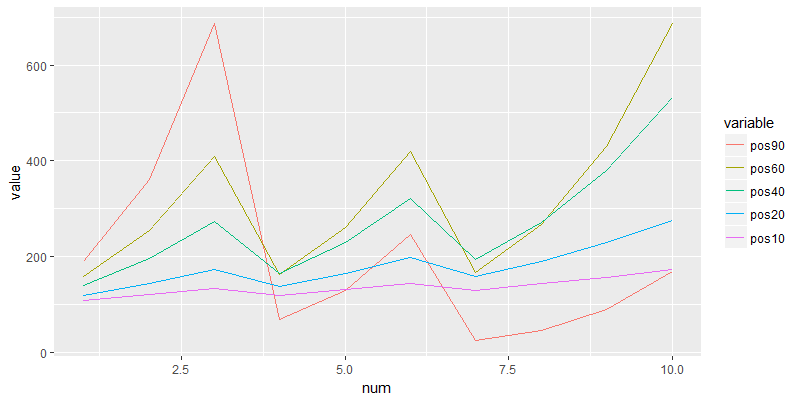## 2. 凯利公式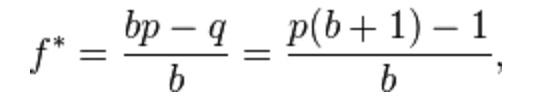• f* 投注的比例
• b 赔率，盈亏比，即平均一次盈利与一次亏损两者的比例
• p 胜率
• q 败率，即 1 – p

``````
f* = (b*p-q)/b = (1*0.8-0.2)/1=0.6
``````

``````
Kelly % = W – [(1 – W) / R]
``````

``````
Kelly % = W – [(1 – W) / R] = 0.8-[(1-0.8)/1] = 0.6
``````

``````
f* =  (b*p-c*q)/c*b
``````

• f* 投注的比例
• b 赔率，盈亏比，即平均一次盈利与一次亏损两者的比例
• p 胜率
• q 败率，即 1 – p
• c 亏损比例

``````
f* = (b*p-q)/b = (1*0.8-0.8*0.2)/(0.8*1)=0.8
``````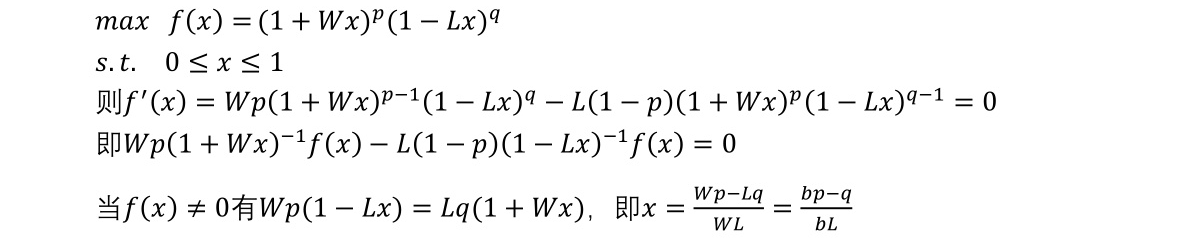## 3. 赌局的最优解

``````
# 凯利公式，实现函数
> kelly<-function(prob,b=1,loss=1){   # 省略代码
+ }
``````

``````
> prob<-0.8                     # 胜率
> b<-1                          # 赔率
> k<-kelly(prob,b,1);k
 0.6
``````

``````
> kelly(0.8,2)
 0.7
``````

``````
> kelly(0.45,2)
 0.175
``````

``````
> kelly(0.45,1)
 "Lost!!!"
 0
``````

``````
> kelly(0.2,1)
 "Lost!!!"
 0
``````

``````
> kelly(0.2,1,0.5)
 "Lost!!!"
 0
``````

``````
> kelly(0.2,1,0.1)
 "All In"
 1
``````

``````
> kelly(0.8,0.1,0.1)
 "All In"
 1
``````

``````
> kelly(0.45,0.1,0.1)
 "Lost!!!"
 0
``````

80% 2 100% 70% 重仓 大胜率、大赔率、全部损失
45% 2 100% 17.5% 轻仓 中胜率、大赔率、全部损失
45% 1 100% 0 离场 中胜率、中赔率、全部损失
20% 1 100% 0 离场 小胜率、中赔率、全部损失
20% 1 50% 0 离场 小胜率、中赔率、中等损失
20% 1 10% 100% 满仓 小胜率、中赔率、小损失
80% 10% 10% 100% 满仓 大胜率、小赔率、小损失
45% 10% 10% 0 离场 中胜率、小赔率、小损失

## 4. 让时间帮我们赚钱

``````
> n<-100                          # 赌局数
> prob<-0.8                       # 胜率
> b<-1                            # 赔率
> caption<-100                    # 金额

# 基本二项分布，生成每盘的赌局正负
> set.seed(1)
> win<-rbinom(n,1,prob)

# 生成每盘的资金
> pos90<-postion(win,b,0.9)*caption   # 90%仓位
> pos60<-postion(win,b,0.6)*caption   # 60%仓位
> pos40<-postion(win,b,0.4)*caption   # 40%仓位
> pos20<-postion(win,b,0.2)*caption   # 20%仓位
> pos10<-postion(win,b,0.1)*caption   # 10%仓位

# 打印数据
> df2<-data.frame(win,pos90,pos60,pos40,pos20,pos10)
> tail(df2)
win     pos90       pos60       pos40   pos20   pos10
95    1 105083487 5.73375e+11  9874948167 5067085 34506.6
96    1 199658625 9.17399e+11 13824927434 6080503 37957.3
97    1 379351388 1.46784e+12 19354898407 7296603 41753.0
98    1 720767637 2.34854e+12 27096857770 8755924 45928.3
99    0  72076764 9.39417e+11 16258114662 7004739 41335.5
100   1 136945851 1.50307e+12 22761360527 8405687 45469.0
``````

``````
# 数据转型
> df2\$num<-1:nrow(df2)
> df<-melt(df2[,-1],id.vars = "num")

# 画图
> g<-ggplot(df,aes(x=num,y=value,colour=variable ))
> g<-g+geom_line()
> g<-g+scale_y_log10()  # y坐标轴log化
> g
``````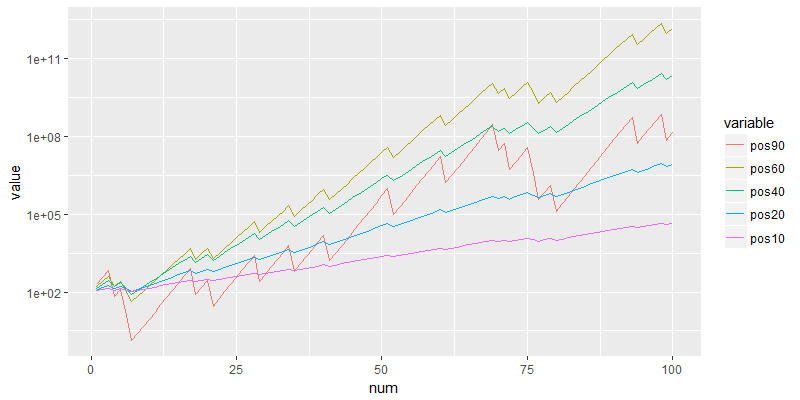``````
> kelly(prob,1)
 0.6
``````This entry was posted in R语言实践, 金融